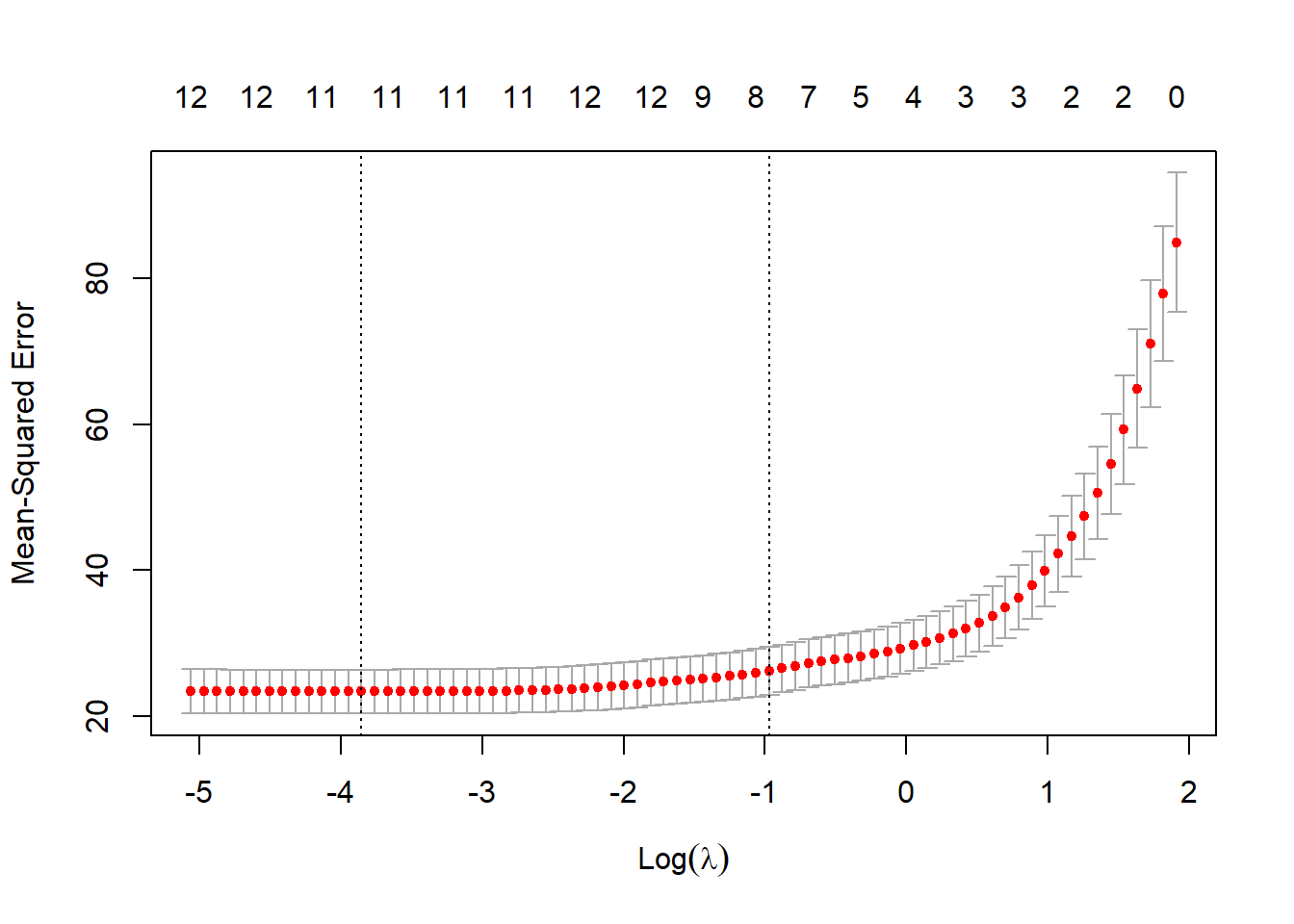# 1 Cross Validation

Cross validation is an alternative approach to training/testing split. For k-fold cross validation, the dataset is divided into k parts. Each part serves as the test set in each iteration and the rest serve as training set. The out-of-sample performance measures from the k iterations are averaged. Instead of fitting a model on a pre-specified 90% training sample and evaluate the MSPE on the hold-out 10% testing sample, it is more reliable to use cross-validation for out-of-sample performance evaluation. For k-fold cross-validation, the dataset is divided into k parts (equal sample size). Each part serves as the testing sample in and the rest (k-1 together) serves as training sample. This training/testing procedure is iteratively performed k times. The CV score is usually the average of the metric of out-of-sample performance across k iterations.

Note

1. We use the entire dataset for cross validation

2. We need to use glm instead of lm to fit the model (if we want to use cv.glm fucntion in boot package)

3. The default measure of performance is the Mean Squared Error (MSE). If we want to use another measure we need to define a cost function.

## 1.1 Cross validation for linear model

### 1.1.1 10-fold Cross Validation

The cv.glm is the cross validation approach in R for glm (more details of arguments are in help doc). By comparing the cross-validation estimate of prediction error, we can tell the full model outperforms the model with only indus and rm in terms of prediction error.

library(MASS)
data(Boston); #this data is in MASS package
library(boot)
model_full <- glm(medv~., data = Boston)
cv.glm(data = Boston, glmfit = model_full, K = 10)$delta ##  24.02774 model_2 <- glm(medv~indus + rm, data = Boston) cv.glm(data = Boston, glmfit = model_2, K = 10)$delta
##  39.70648

### 1.1.2 10-fold Cross Validation Using MAE

Here we need to define a MAE cost function. The function takes 2 input vectors, pi = predicted values, r = actual values.

MAE_cost <- function(pi, r){
return(mean(abs(pi-r)))
}

cv.glm(data = Boston, glmfit = model_full, cost = MAE_cost, K = 10)$delta ##  3.368605 cv.glm(data = Boston, glmfit = model_2, cost = MAE_cost, K = 10)$delta
##  4.274093

### 1.1.3 LOOCV (Leave-one-out Cross Validation)

The same finding is observed by conducting LOOCV.

cv.glm(data = Boston, glmfit = model_full, K = nrow(Boston))$delta ##  23.72388 cv.glm(data = Boston, glmfit = model_2, K = nrow(Boston))$delta
##  39.94028

### 1.1.4 Cross Validation for search optimal tuning parameter in LASSO

Using 10-fold cross-validation to search optimal lambda:

library(glmnet)
lasso_fit  <- glmnet(x = as.matrix(Boston[, -c(which(colnames(Boston)=='medv'))]), y = Boston$medv, alpha = 1) #use 10-fold cross validation to pick lambda cv_lasso_fit = cv.glmnet(x = as.matrix(Boston[, -c(which(colnames(Boston)=='medv'))]), y = Boston$medv, alpha = 1, nfolds = 10)
plot(cv_lasso_fit)The best $$\lambda$$ (or s) is given by:

cv_lasso_fit$lambda.min ##  0.02118502 Given a selected s you can use predict() this way to get prediction: coef(lasso_fit, s = cv_lasso_fit$lambda.min)
## 14 x 1 sparse Matrix of class "dgCMatrix"
##                         1
## (Intercept)  34.880894908
## crim         -0.100714832
## zn            0.042486737
## indus         .
## chas          2.693903097
## nox         -16.562664327
## rm            3.851646315
## age           .
## dis          -1.419168850
## tax          -0.010286456
## ptratio      -0.933927773
## black         0.009089735
## lstat        -0.522521473
pred_IS <- predict(lasso_fit, as.matrix(Boston[, -c(which(colnames(Boston)=='medv'))]), s = cv_lasso_fit$lambda.min) MAE_cost(pred_IS, Boston$medv)
##  3.259372

### 1.1.5 (Optional) Supplementary package: DAAG

Another package DAAG also does cross validation. It prints out the performance in each fold and gives you a plot at the end. But currently I cannot figure out how to get the cross-validation error programmatically.

install.packages('DAAG')
library(DAAG)
model_2 <- lm(medv~indus + rm, data = Boston)
cv3 <- cv.lm(data = Boston, form.lm = model_2, m=3)

go to top